# 978-0123869449 Chapter 11 Part 2

Document Type
Homework Help
Book Title
Authors
Michael F. Modest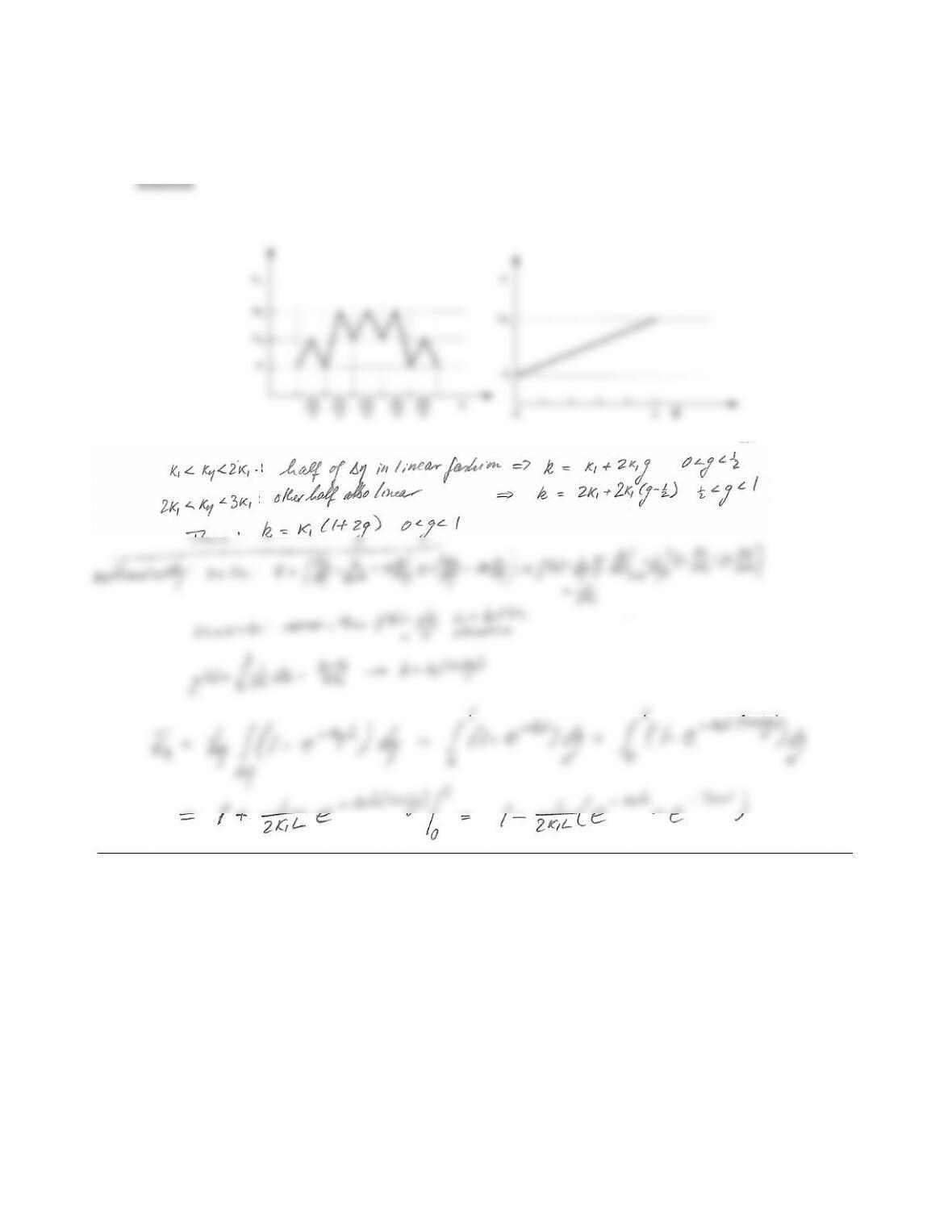CHAPTER 11 309
11.20 Consider the spectral absorption coecient for a narrow band range of ηas given by the sketch. Carefully
sketch the corresponding k-distribution. Determine the mean narrow band emissivity of a layer of thickness
Lfrom this k-distribution.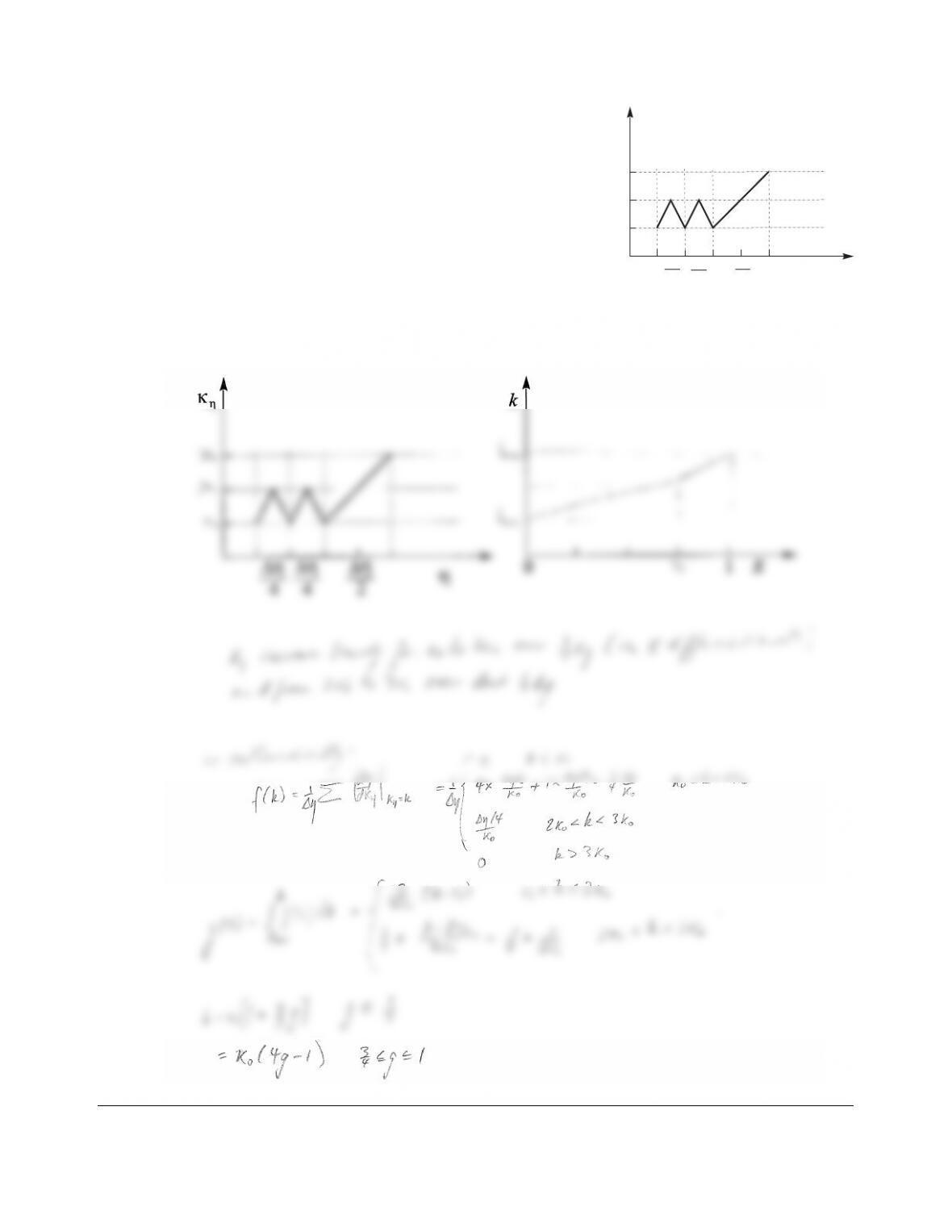11.21
κ
η
η
∆η
4
∆η
4
∆η
2
1
2 1
3 1
κ
κ
κ
Consider the spectral absorption coecient for a narrow band
range of ηas given by the sketch. Carefully sketch the corre-
sponding k-distribution. Verify your sketch through calculations.
Solution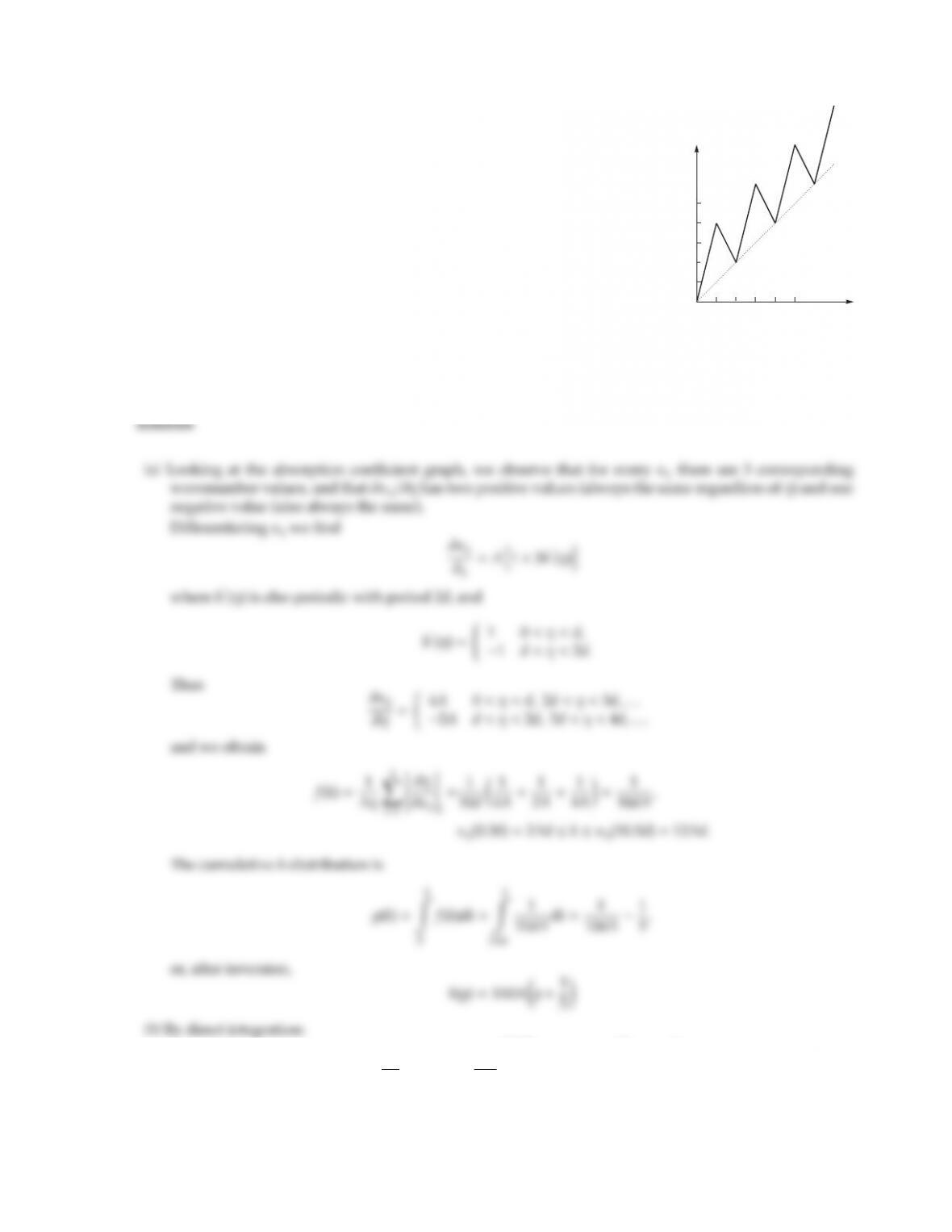CHAPTER 11 311
11.22
κη
η
d4d
Consider the (highly artificial) absorption coecient shown. Mathemati-
cally, this may be expressed as
κη=A[η+3h(η)] 0 < η <
where h(η) is a periodic function of period 2d, defined by
h(η)=η, 0< η < d,
2dη, d< η < 2d.
For a narrow band of η=10dand 0.5d< η < 10.5d,
(a) find the k-distribution as well as the cumulative k-distribution,
(b) determine the narrow band average absorption coecient ¯
κηby direct (η) integration, and also from
the k-distribution,
(c) determine the narrow band average transmissivity for a slab of thickness Lfrom the k-distribution;
compare with the gray-gas value (using ¯
κη),
¯
κη=1
ηZ
η
κηdη=1
10d
10.5d
Z
.5d
ηdη+5×3
2d
Z
0
h(η)dη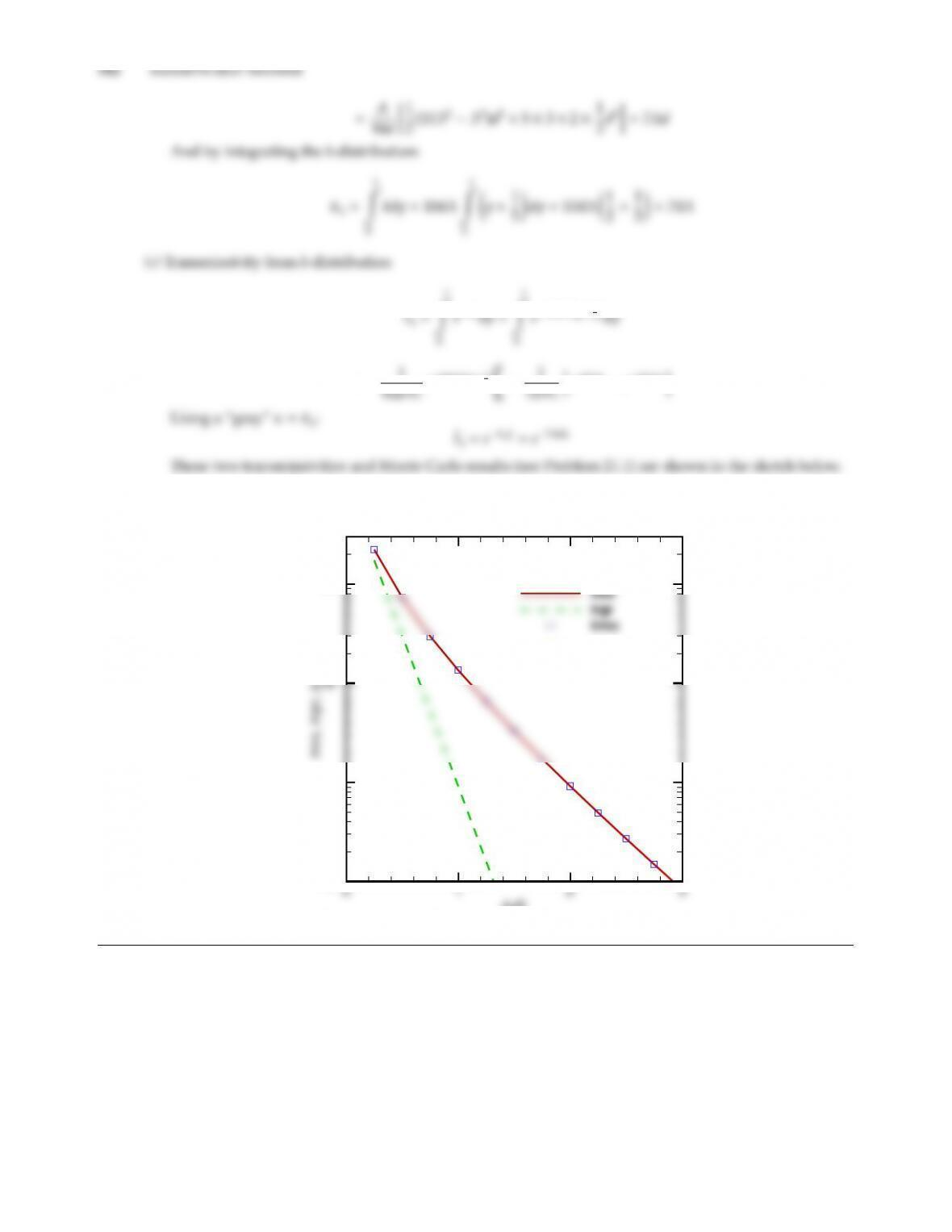=1
5)
=1
10-4
10-3
10-1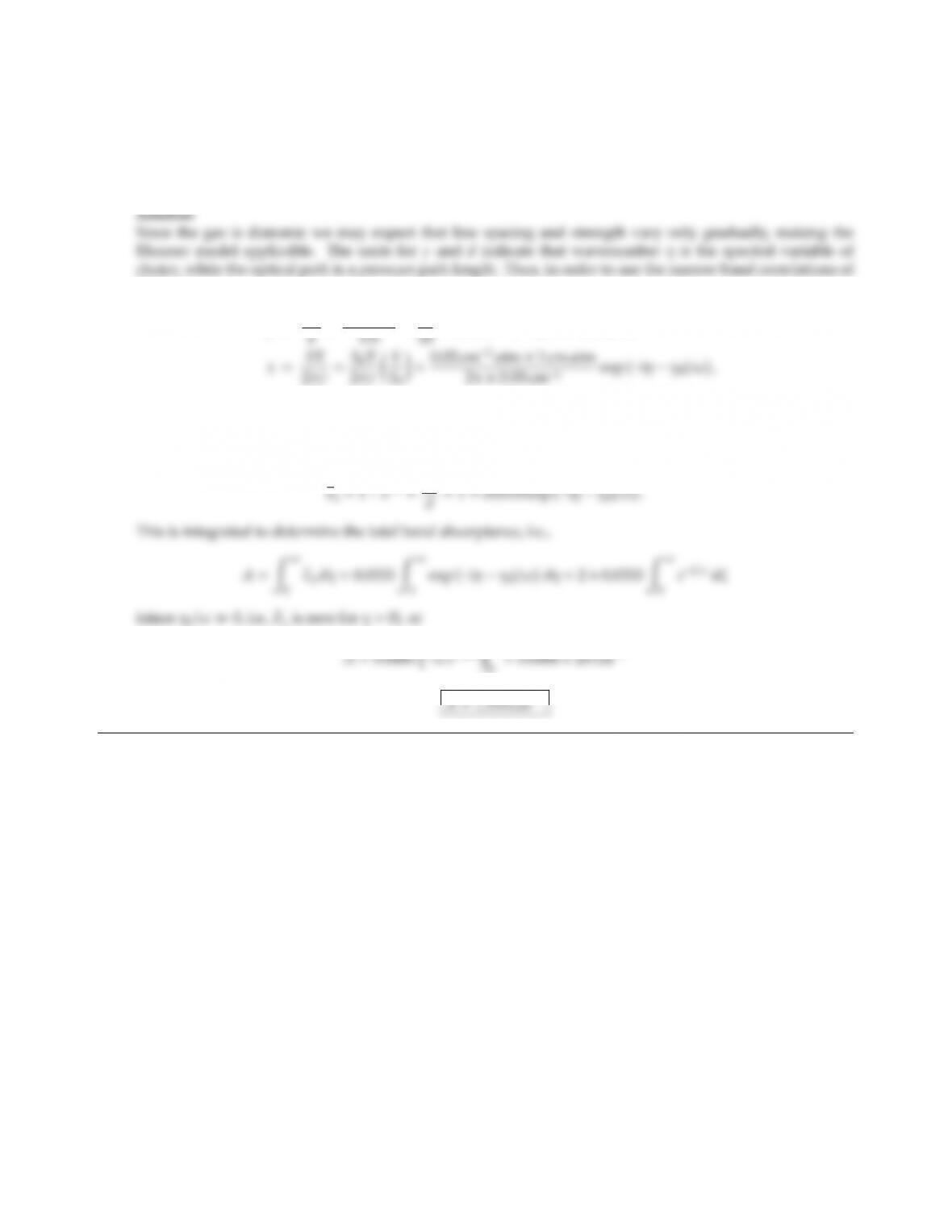CHAPTER 11 313
11.23 Consider again the gas of Problem 11.15, but replace the line intensity by
Spη=Spη0e−|ηη0|,Spη0=0.02 cm2atm1, ω =20 cm1.
In what regime is this optical condition? What is the total band absorptance?
Table 11.1,
β=πγ
x=0.106 exp −|ηη0|, τ =2βx=0.0333 exp −|ηη0|.
Thus we are, at the band center, in the weak line regime as well as in the non-overlap regime; this is even
more true for spectral locations away from the band center. Thus, from Table 11.1
0=0.0666 ×20 cm1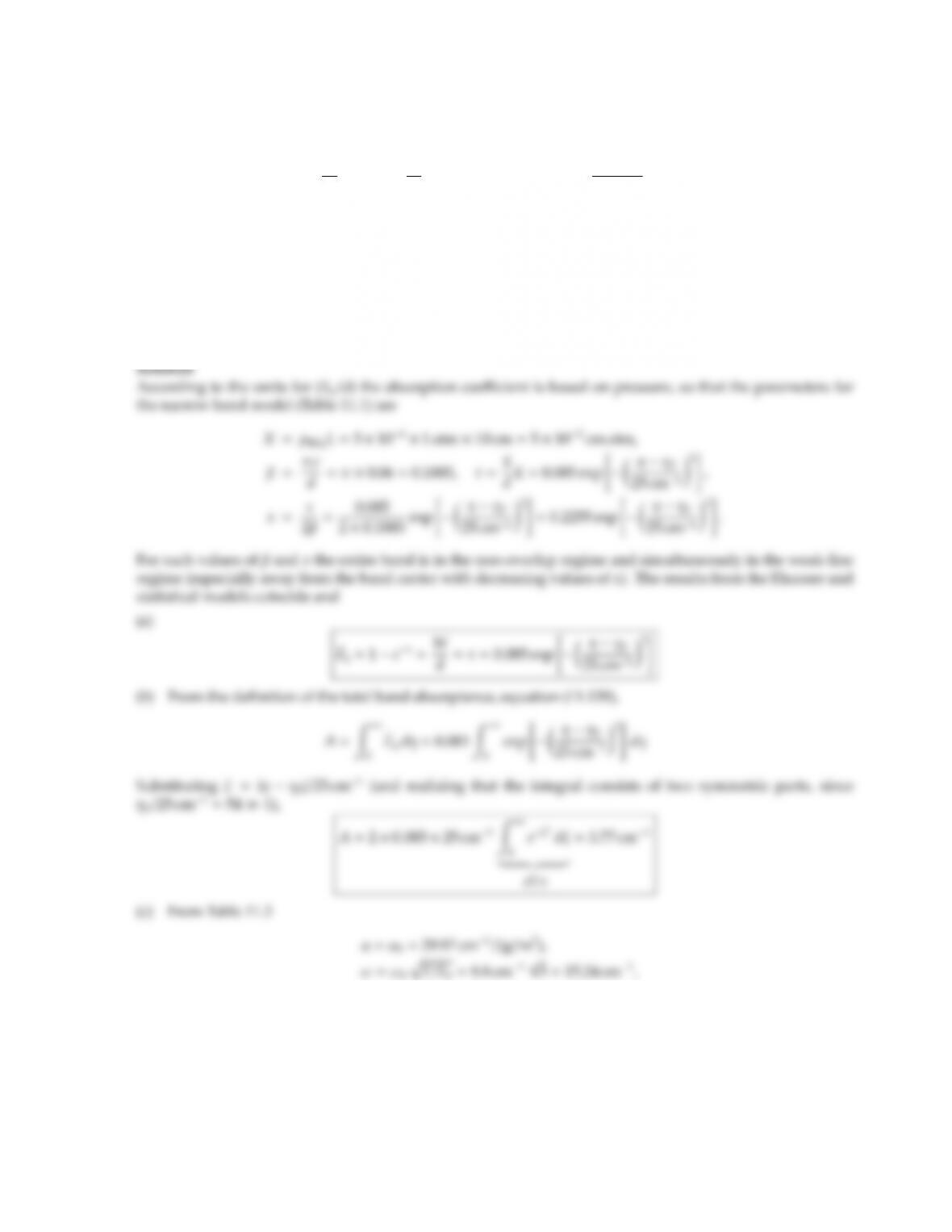11.24 A mixture of nitrogen and sulfur dioxide (with 0.05 volume-% SO2) is at 1 atm and 300 K. For the strong
ν3-band of SO2, centered at η3=1361 cm1, it is known that
γη
d0.06,Sη
d=17 cm1atm1exp "ηη3
25 cm12#.
For a 10 cm thick gas-mixture layer:
(a) Develop an expression for the average spectral emissivity. What regime(s) apply?
(b) Calculate the total band absorptance.
(c) For comparison, calculate the total band absorptance from the wide band model. (Hint: For 300 K
β
0>1).
n=0.65,b=1.28.
Thus,
Pe=1+0.25 ×5×1040.65 1.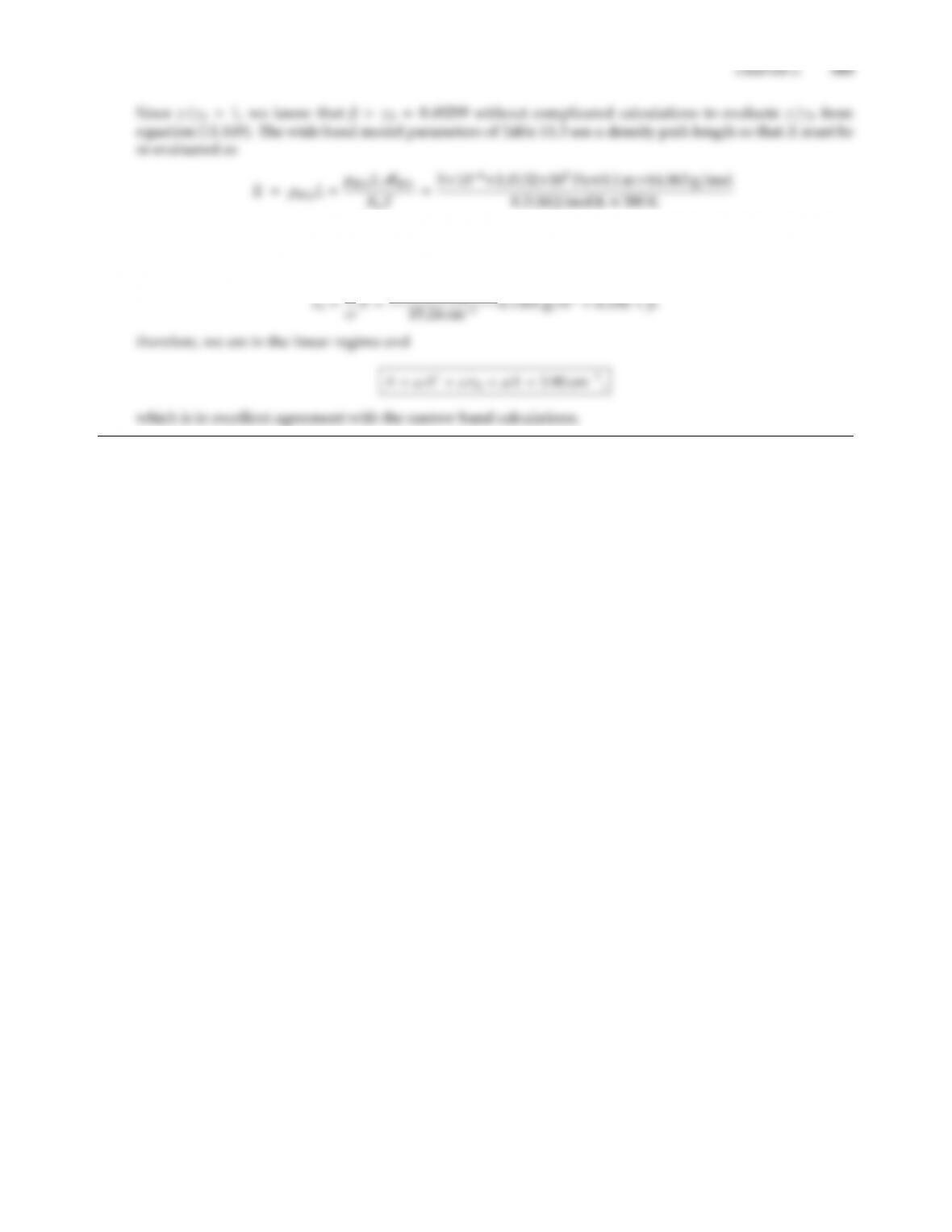=0.1301 g/m2.
Thus,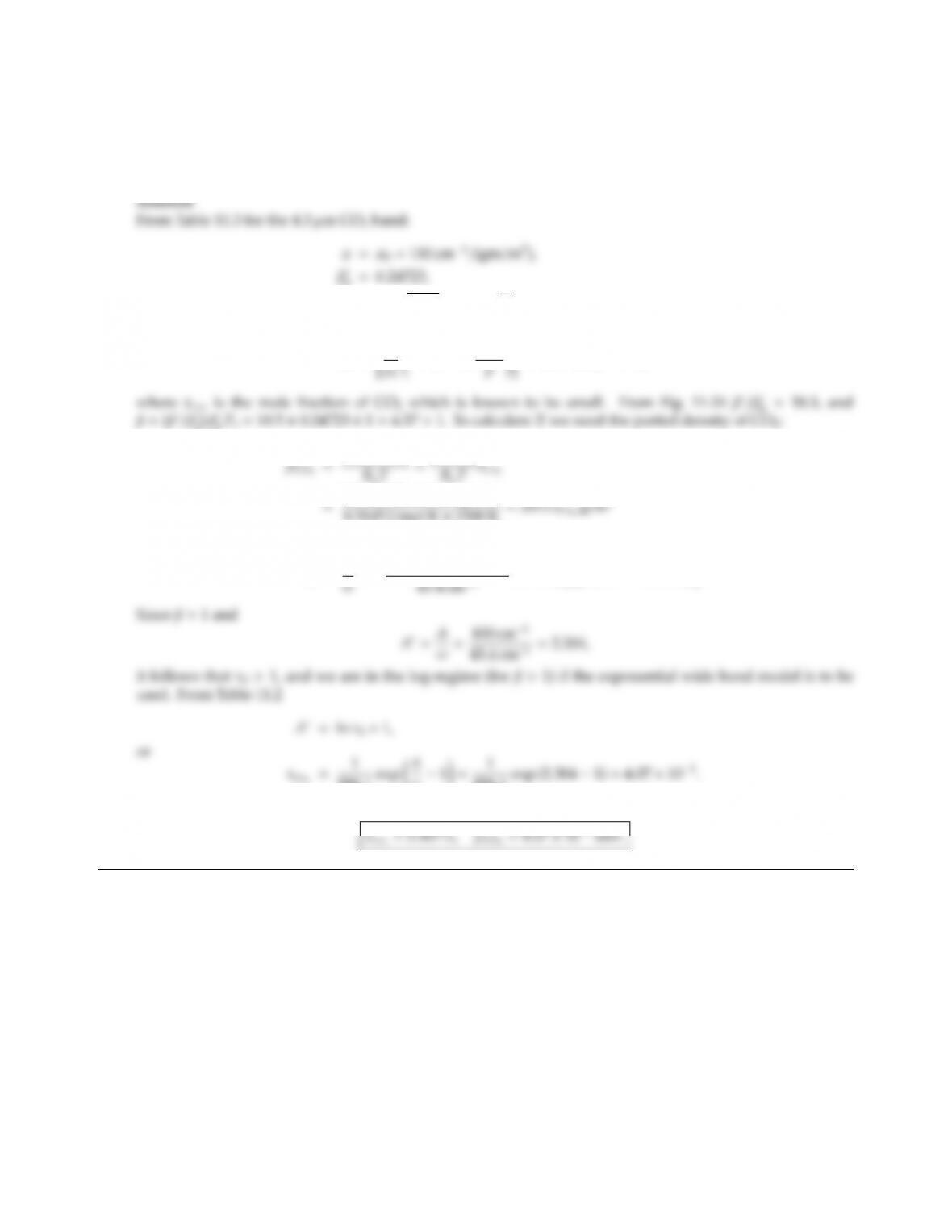11.25 A gas mixture at 1500 K and 1 atm is known to contain a small amount of CO2. To remotely determine the
partial pressure of CO2the band absorptance of the CO24.3µm band is measured and is found to be 100 cm1
for a path length of 1 m. Assuming that the gas may be treated as a nitrogen–CO2mixture, determine the
partial pressure of the CO2.
0=0.24723,
ω=ω0pT/T0=11.215 =43.4 cm1,
n=0.8,b=1.3,
Pe="p
=(1 +0.3x)0.81,
0)β
0Pe=18.5×0.24723 ×1=4.57 >1. To calculate Xwe need the partial density of CO2:
8.3145 J/mol K ×1500 K =3575 xCO2g/m3
X=ρCO2L=357.5xCO2gm/m2,
τ0=α
906.1exp A
ω1=1
906.1exp (2.304 1)=4.07 ×103.
Thus,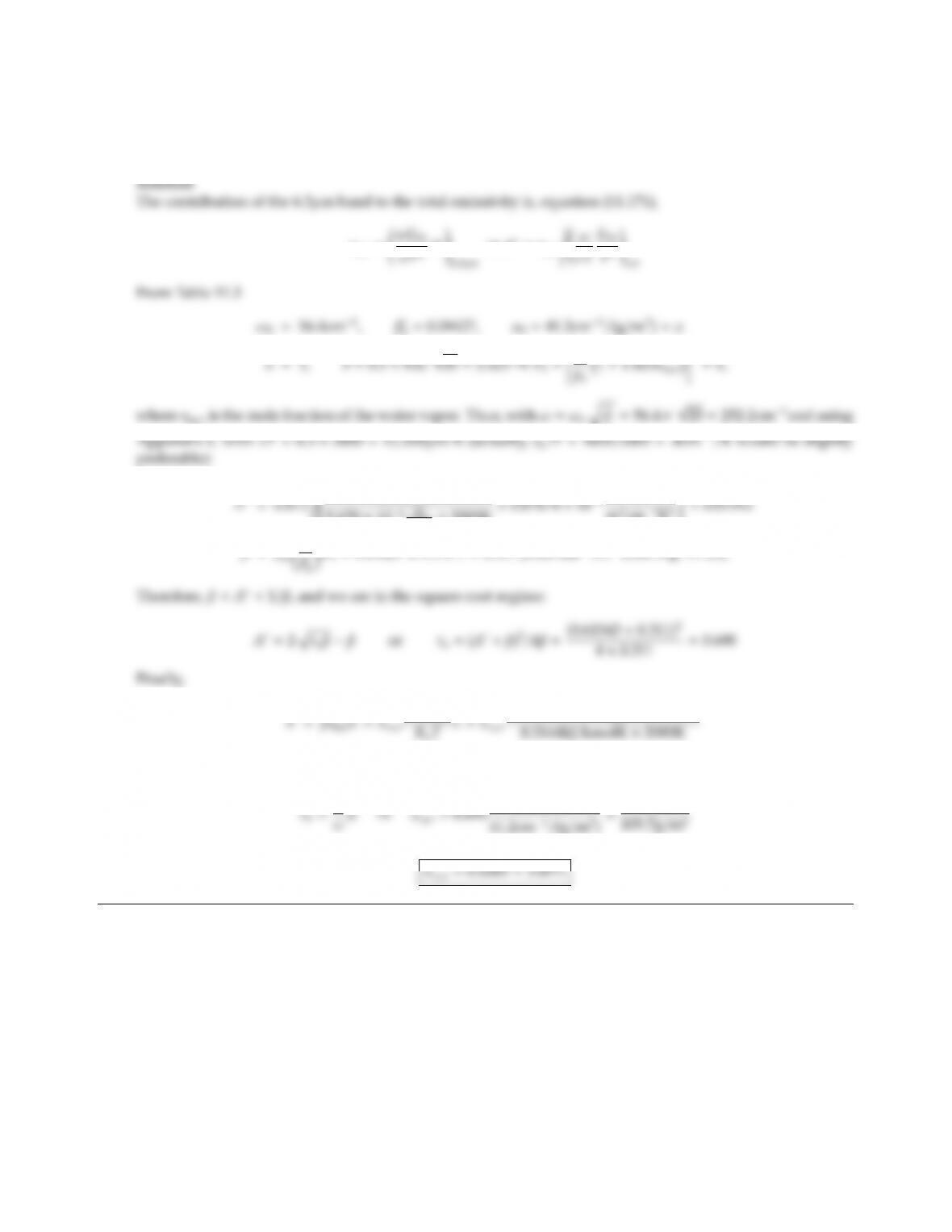CHAPTER 11 317
11.26 Nitrogen at 2000 K and 1 atm contains a small amount of water vapor. To remotely determine the water vapor
concentration the contribution of the 6.3 µm band toward the total emissivity is measured, and is found to be
ǫ6.3=0.012 for a 1 m thick isothermal gas layer. Determine the mole fraction of water vapor.
ǫ6.3= ωEbη
or A=ǫ6.3, ω
0=0.09427, α0=41.2cm1/(g/m2)=α
To=56.4×20 =252.2cm1and using
252.2cm1
5.670 ×108W
m2K4×2000K ×0.87674 ×108W
m2cm1K3
β=β
pMH2O
101.32kPa ×18kg/kmol ×1m
=109.7xH2Og/m2
and
and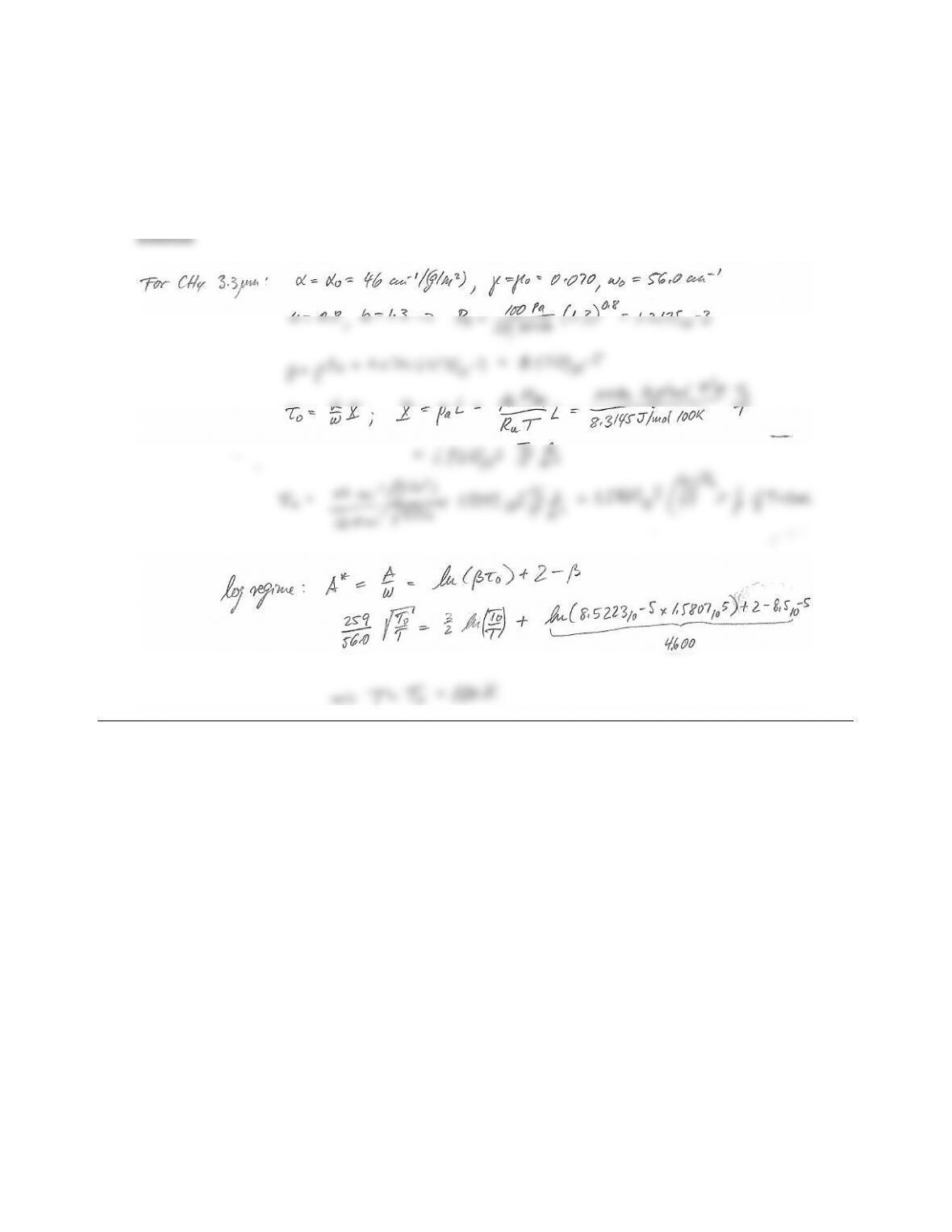11.27 A large moon in the outer solar system has a thin atmosphere of pure methane. In order to determine average
temperatures of the atmosphere, a satellite measures the total band absorptance of the 3.3 µm fundamental
band at an altitude where the atmospheric pressure is 100Pa. If the total band absorptance for a 100 km thick
layer is measured as A3.3=259 cm1, determine the temperature of the atmosphere (this may be left in the
form of an implicit expression).
Note: at the expected cold temperature (<200K) you may assume γγ0,γ(T).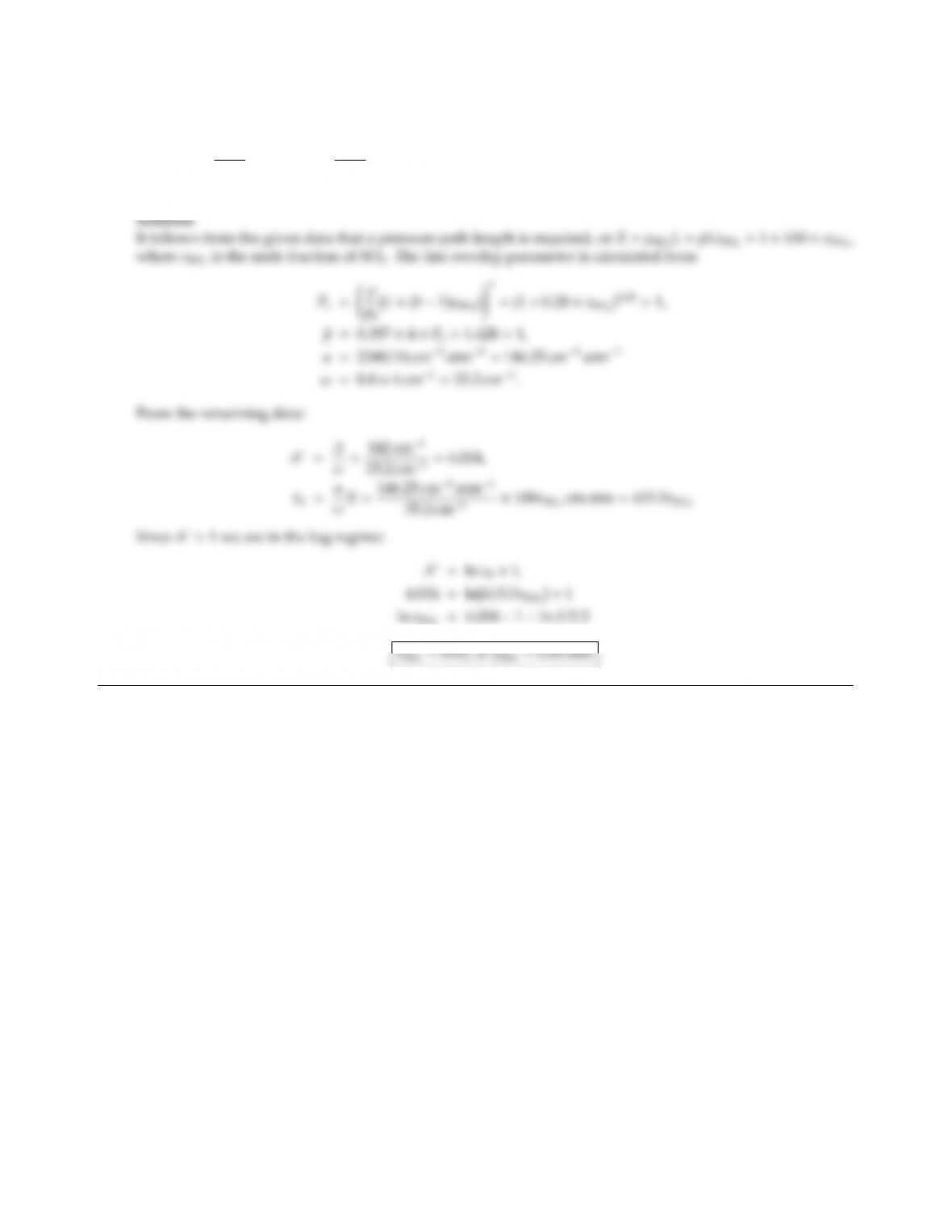CHAPTER 11 319
11.28 A mixture of nitrogen and sulfur dioxide is at 1 atm total pressure. To measure the partial pressure of the
sulfur dioxide in a furnace environment, an instrument is used that measures total band absorptance for
the strong SO2-band centered at ηc=1361 cm1. For that band it is known that α=2340 (T0/T) cm2atm1,
β=0.357 T/T0Pe,ω=8.8T/T0cm1,b=1.28 and n=0.65. What is the partial pressure of the sulfur
dioxide if the total band absorptance has been measured as 142 cm1for a 1 m thick gas layer at T=1600 K?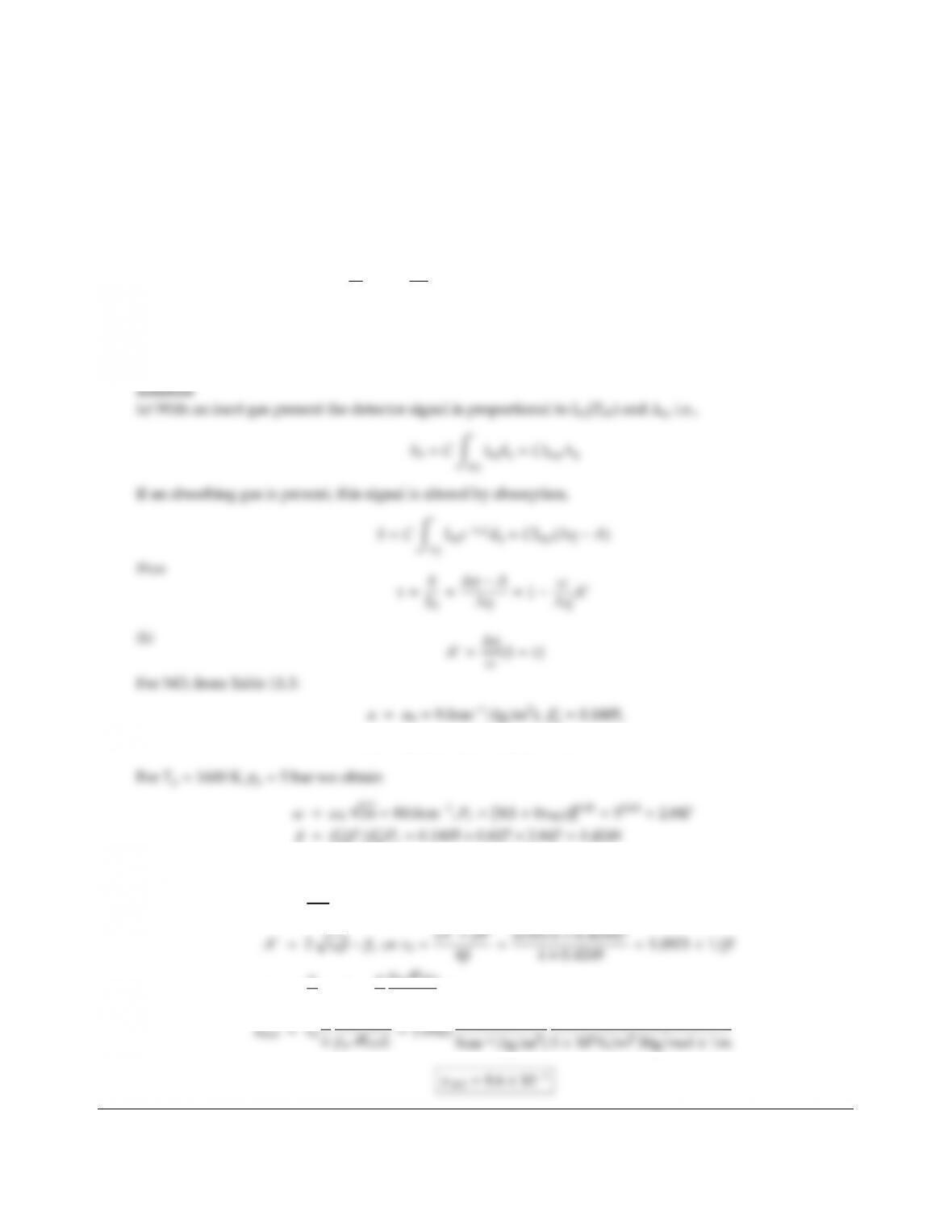11.29 An optical device to determine NOxcontent in the combustion products powering a gas turbine measures the
transmissivity of NO for its 5.3 µm band. The device consists of a blackbody source (Tbb =2000 K), two ports,
a filter/detector combination (with flat response 1750 cm1< η < 2000 cm1, and zero response elsewhere),
and a chopper (such that the detector registers radiation from the blackbody source, but not the emission from
the combustion gases). Two measurements are taken: one with an inert gas in the turbine (signal S0), and
another with combustion gases present (assume T1=1600 K =const, p1=5 bar =const, light path through
gas L=1 m; signal S).
(a) Show that the measured transmissivity, τ, is
τS
S0
=1ω
ηA(T1,p1,L,xNO),η=250cm1,
assuming that the Planck function is constant across η.
(b) If the transmissivity is measured as τ=0.7, what is the NO volumetric concentration, xNO?
(Note: for NO at 1600 K β
0=0.827).
0=0.1805,
ω0=20.0cm1,n=0.65,b=1.0
0(β
0)Pe=0.1805 ×0.827 ×2.847 =0.4249
Thus
A=250
80 (1 0.7) =0.9375 <1=probably square root–regime
τ0=α
ωρNOL=α
ω
p1MNO
RuTL xNO
ω
RuT1
8.314 ×1600J/mol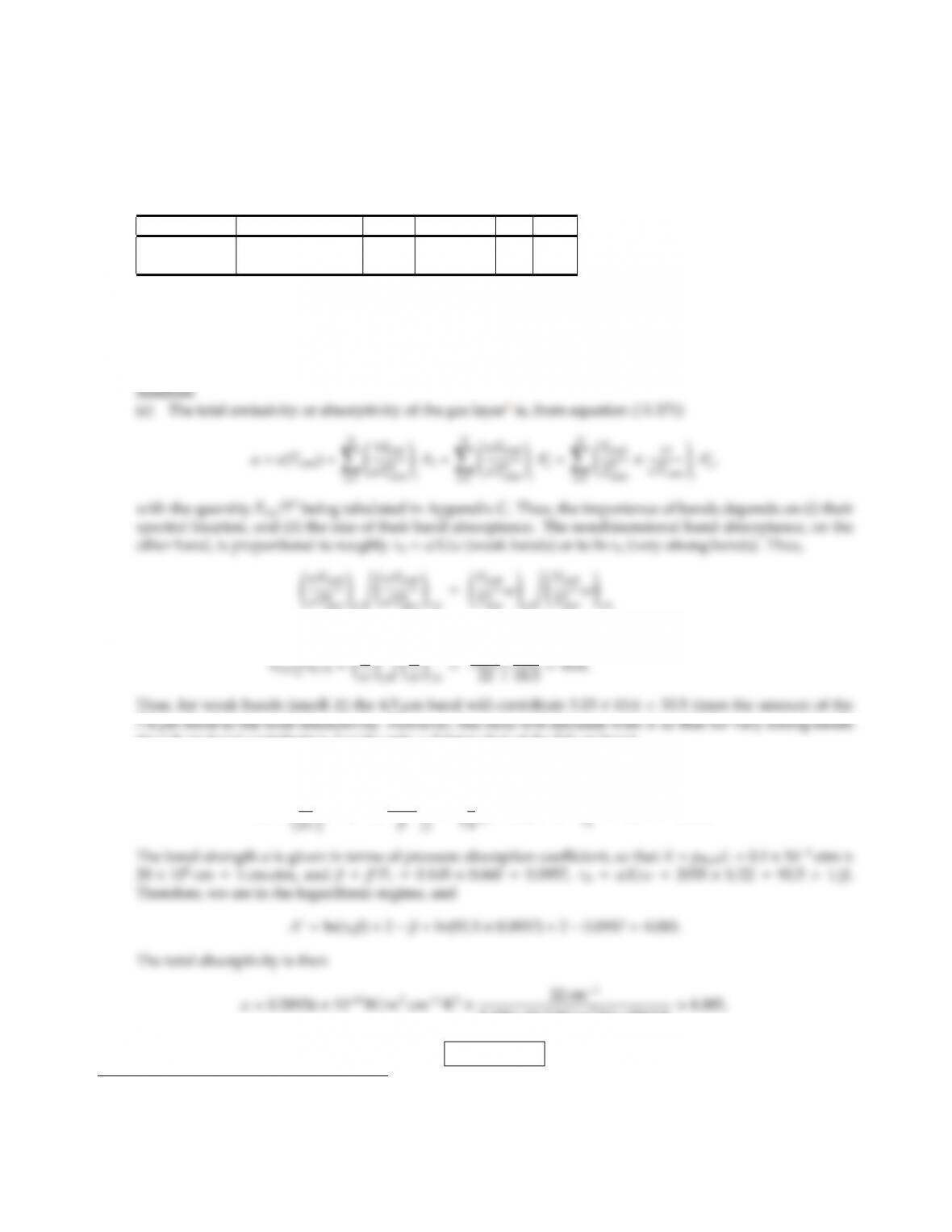CHAPTER 11 321
11.30 It is desired to predict the fraction of the sunlight absorbed by the nitrous oxide (N2O) contained in the
atmosphere. You may assume that the atmosphere is a 20 km high layer of N2–N2O mixture, that the
atmosphere is isothermal with a linear pressure variation from 1 atm at the ground to zero at the top of the
atmosphere, and that N2O makes up 104% by volume of the mixture everywhere.
N2O has two vibration-rotation bands with the following wide band coecients (at the temperature of the
atmosphere):
α βωn b
4.5µm band 2035 cm2atm10.145 22 cm10.6 1.12
7.8µm band 161 cm2atm10.377 18.5 cm10.6 1.12
(a) Show that the influence of the 7.8µm band is negligible.
(b) Calculate the total absorptivity for this atmosphere assuming a constant average pressure of 0.5 atm.
(c) Show that the absorptivity is the same as in (b) if the linear pressure variation is taken into account. Hint:
You may assume that the pressure-absorption coecient, κpη, is independent of pressure.
=(0.28926 ×22)/(0.10920 ×18.5) =3.15,
the 4.5µm band contribution may be only 5 times that of the 7.8µm band.
(b) To calculate the band absorptance from Table 11.2, we need to establish X,τ0and βfor the 4.5µm band:
Pe=(p
=1
0.50.6=0.660.
5.670×108W/m2K4×5762 K ×4.085,
α=8.0×104
1Note that in this problem absorptivity and band strength are both denoted by the letter α.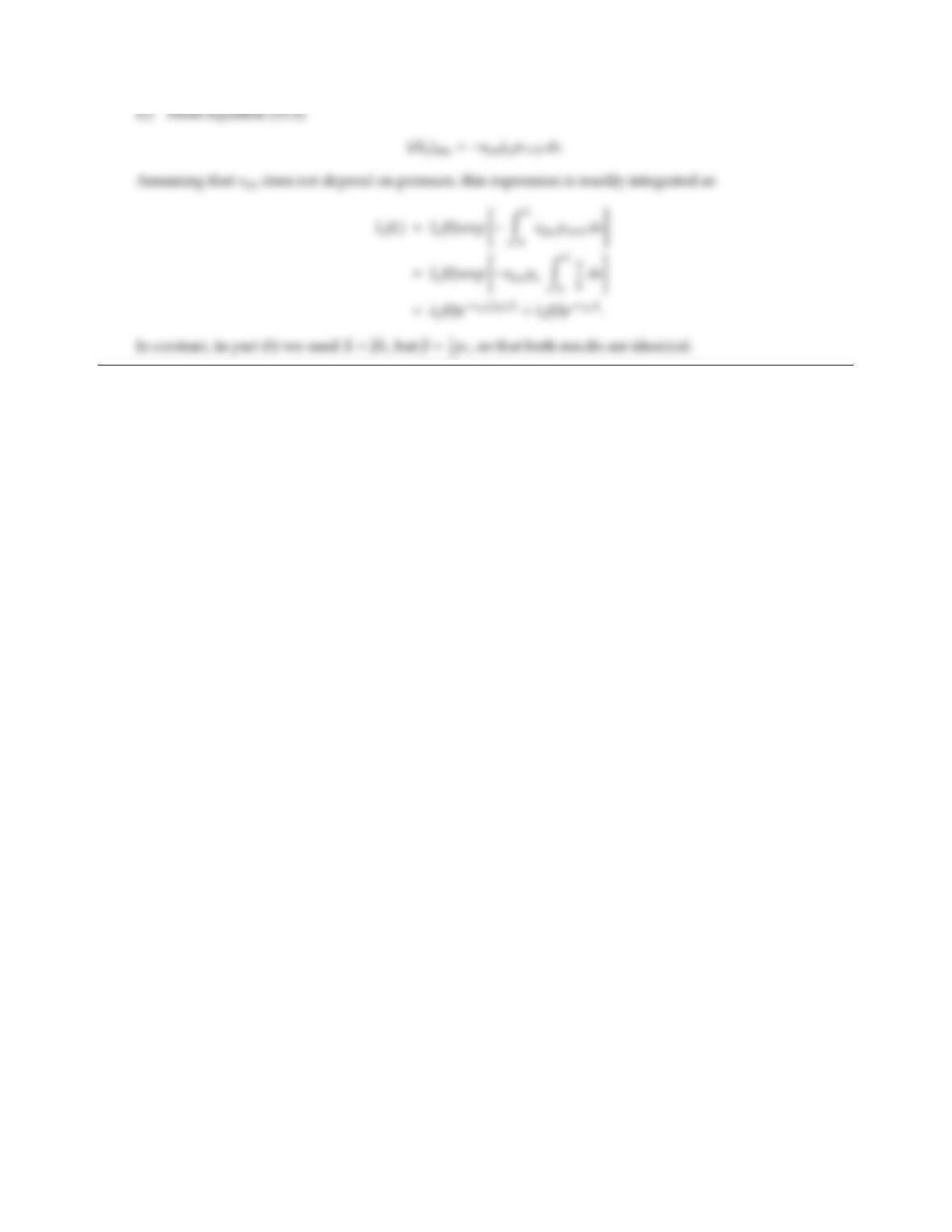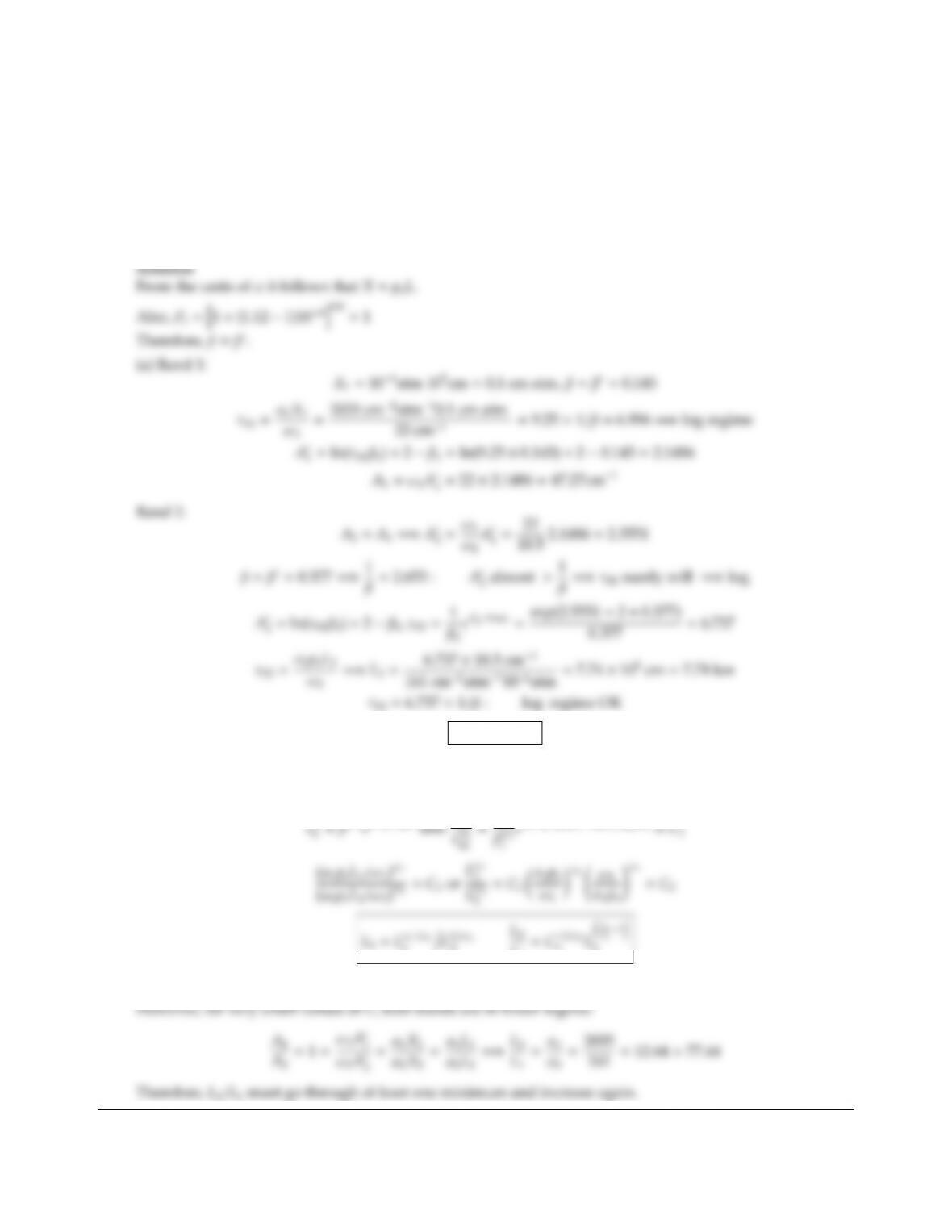CHAPTER 11 323
11.31 Nitrous oxide (N2O) is contained in the earth’s atmosphere with a partial pressure of 106atm. While N2O
causes pollution, it may also be employed for remote sensing applications. N2O has two vibration-rotation
bands with the wide band coecients (at the temperature of the atmosphere) given in Problem 11.30.
(a) It is known that, for a distance of L1=1 km through the atmosphere, the 4.5 µm band has a certain total
band absorptance A1. What length through the atmosphere, L2, is required to obtain the same band
absorptance with the 7.8 µm band, A2=A1?
(b) Would this ratio L2/L1increase, decrease, or stay the same if L1was decreased?
L2=7.74 km
b) If L1is decreased a little both bands will stay in log regime.
Raising expression for τ0to power ω(and A=ωA)
01
2
1.C12
2
L1
2Lω1
1
Since ω1210.1970 as L1decreases so does L2/L1.

## Trusted by Thousands ofStudents

Here are what students say about us.